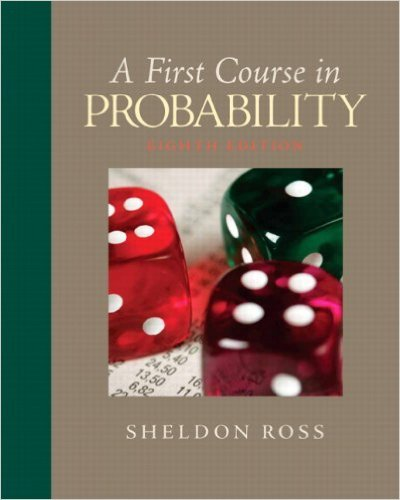×

×

# Solutions for Chapter 1: Combinatorial Analysis## Full solutions for First Course in Probability | 8th Edition

ISBN: 9780136033134Solutions for Chapter 1: Combinatorial Analysis

Solutions for Chapter 1
4 5 0 303 Reviews
25
5
##### ISBN: 9780136033134

Summary of Chapter 1: Combinatorial Analysis

This textbook survival guide was created for the textbook: First Course in Probability, edition: 8. Since 33 problems in chapter 1: Combinatorial Analysis have been answered, more than 26471 students have viewed full step-by-step solutions from this chapter. First Course in Probability was written by and is associated to the ISBN: 9780136033134. This expansive textbook survival guide covers the following chapters and their solutions. Chapter 1: Combinatorial Analysis includes 33 full step-by-step solutions.

Key Statistics Terms and definitions covered in this textbook

A formula used to determine the probability of the union of two (or more) events from the probabilities of the events and their intersection(s).

• Attribute control chart

Any control chart for a discrete random variable. See Variables control chart.

• Backward elimination

A method of variable selection in regression that begins with all of the candidate regressor variables in the model and eliminates the insigniicant regressors one at a time until only signiicant regressors remain

• Biased estimator

Unbiased estimator.

• Box plot (or box and whisker plot)

A graphical display of data in which the box contains the middle 50% of the data (the interquartile range) with the median dividing it, and the whiskers extend to the smallest and largest values (or some deined lower and upper limits).

• Categorical data

Data consisting of counts or observations that can be classiied into categories. The categories may be descriptive.

• Completely randomized design (or experiment)

A type of experimental design in which the treatments or design factors are assigned to the experimental units in a random manner. In designed experiments, a completely randomized design results from running all of the treatment combinations in random order.

• Conidence coeficient

The probability 1?a associated with a conidence interval expressing the probability that the stated interval will contain the true parameter value.

• Contrast

A linear function of treatment means with coeficients that total zero. A contrast is a summary of treatment means that is of interest in an experiment.

• Correlation

In the most general usage, a measure of the interdependence among data. The concept may include more than two variables. The term is most commonly used in a narrow sense to express the relationship between quantitative variables or ranks.

• Crossed factors

Another name for factors that are arranged in a factorial experiment.

• Discrete random variable

A random variable with a inite (or countably ininite) range.

• Empirical model

A model to relate a response to one or more regressors or factors that is developed from data obtained from the system.

• Error propagation

An analysis of how the variance of the random variable that represents that output of a system depends on the variances of the inputs. A formula exists when the output is a linear function of the inputs and the formula is simpliied if the inputs are assumed to be independent.

• Factorial experiment

A type of experimental design in which every level of one factor is tested in combination with every level of another factor. In general, in a factorial experiment, all possible combinations of factor levels are tested.

• First-order model

A model that contains only irstorder terms. For example, the irst-order response surface model in two variables is y xx = + ?? ? ? 0 11 2 2 + + . A irst-order model is also called a main effects model

• Forward selection

A method of variable selection in regression, where variables are inserted one at a time into the model until no other variables that contribute signiicantly to the model can be found.

• Fraction defective control chart

See P chart

• Gamma random variable

A random variable that generalizes an Erlang random variable to noninteger values of the parameter r

• Goodness of fit

In general, the agreement of a set of observed values and a set of theoretical values that depend on some hypothesis. The term is often used in itting a theoretical distribution to a set of observations.